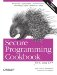# Using Pointers to Class Members

Problem

You need to refer to a data member or a member function with its address.

Solution

Use the class name and the scope operator (::) with an asterisk to correctly qualify the name. Example 15-2 shows how.

Example 15-2. Obtaining a pointer to a member

```#include
#include

class MyClass {

public:
MyClass( ) : ival_(0), sval_("foo") {}
~MyClass( ) {}

void incr( ) {++ival_;}
void decr( ) {ival_--;}

private:
std::string sval_;
int ival_;
};

int main( ) {

MyClass obj;

int MyClass::* mpi = &MyClass::ival_; // Data member
std::string MyClass::* mps = &MyClass::sval_; // pointers

void (MyClass::*mpf)( ); // A pointer to a member function that
// takes no params and returns void
void (*pf)( ); // A normal function pointer

int* pi = &obj.ival_; // int pointer referring to int member--no
// problem.

mpf = &MyClass::incr; // A pointer to a member function. You can't
// write this value to a stream. Look at it
// in your debugger to see what its
// representation looks like.

pf = &MyClass::incr; // Error: &MyClass::incr is not an instance
// of a function

std::cout << "mpi = " << mpi << '
';
std::cout << "mps = " << mps << '
';
std::cout << "pi = " << pi << '
';
std::cout << "*pi = " << *pi << '
';

obj.*mpi = 5;
obj.*mps = "bar";

(obj.*mpf)( ); // now obj.ival_ is 6

std::cout << "obj.ival_ = " << obj.ival_ << '
';
std::cout << "obj.sval_ = " << obj.sval_ << '
';
}```

Discussion

Pointers to members look and act differently than ordinary pointers. First of all, they have funny syntax (not funny ha-ha, funny strange). Consider the following line, from Example 15-2:

`int MyClass::* mpi = &MyClass::ival_;`

This declares and assigns a pointer to an integer that happens to be a member of the class MyClass. Two things make this different than an ordinary int*. First, you have to include the class name and the scope operator in between the data type and the asterisk. Second, when you assign this pointer, you aren't actually assigning it the address of something in memory. The value &MyClass::ival_ is not a concrete value in memoryit can't be; it refers to the class name, not an object namebut what is it? Think of it as an offset of the data member from the object's start address.

The variable mpi has to be used with an instance of the class to which it applies. A little further down in Example 15-2, this line uses mpi to assign an integer to the value pointed to by mpi:

`obj.*mpi = 5;`

obj is an instance of the class MyClass. By referring to the member using the dot notation (or -> if you have a pointer to obj) and dereferencing mpi, you get a reference to obj.ival_.

Pointers to member functions are essentially the same. Example 15-2 declares a pointer to a member function of MyClass that returns void and takes no arguments:

`void (MyClass::*mpf)( );`

Assign it to a value with the address-of operator:

`mpf = &MyClass::incr;`

To invoke it, place parenthesis around the main expression to ensure the compiler knows what you're doing, like this:

`(obj.*mpf)( );`

There is one difference in data member pointers and function pointers though. If you want to point an ordinary, nonmember pointer at a data member, just do it as you would expect:

`int* pi = &obj.ival_;`

Of course, you use an object name and not a class name, because you are getting the address of the concrete data member of a specific object somewhere in memory. (Typically, though, you don't want to give out addresses to your class's data members, lest they be inadvertently changed by reckless client code.)

By contrast, you can't do the same thing with a member function because it makes no sense. Consider a function pointer that assumes the same function signature as MyClass::incr (i.e., returns void and takes no arguments):

`void (*pf)( );`

Now, try to assign the address of a member function to it:

```pf = &MyClass::incr; // Nope
pf = &obj.incr; // No dice```

Neither of these will compile, and for good reason. A member function requires an object context to make any sense, since it most likely refers to member variables. Invoking a member function without an object would require that the member function not use any of the object's members, which is presumably why it's a member function and not a standalone function.

Recipe 15.1Secure Programming Cookbook for C and C++: Recipes for Cryptography, Authentication, Input Validation & More
ISBN: 0596003943
EAN: 2147483647
Year: 2006
Pages: 241